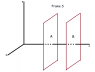# Special Relativity Simple Problem

• IDumb
Yarbrough, a student, is struggling with homework. He asks for help on the Homework Forum and posts a question about understanding what the velocity of the flash is. He does not understand what a hyper surface is or how to look at the problem in two dimensions only. He asks for help with understanding the problem and the help he gets is attached to the post.

## Homework Statement

Consider the usual two inertial frames S and S' in standard configuration. In S' the standard lattice clocks all emit a 'flash' at noon. Prove that in S this flash occurs on a plane orthogonal to the x-axis and traveling in the positive x-direction at (de-Broglie-) speed c^2/v.

## Homework Equations

Lorentz transforms. The invariant interval (ct^2 - x^2 - y^2 - z^2 = ct'^2 - x'^2 - y'^2 - z'^2). To clarify standard configuration is just an orthogonal x y z for s and then an orthogonal x' y' for s' where x and y are parallel to x' and y' respectively and s' is moving away from s at speed v along the x and x' axis.

## The Attempt at a Solution

I'm struggling to begin. I think I'm misunderstanding something... because in thinking about limiting cases. Say we have an S' frame that happens to be the same as S (so v = 0), then the problem makes no sense. I feel like in that case the flash should be moving at a speed of 0. Perhaps this is misunderstanding what it means by a "flash occurring on a plane".

I just really need a hint on how to get started on this : (.

If v = 0, the flash is happening everywhere at once and thus, in some sense, travels with infinite speed (the time between reaching x = 0 and x = 2000 lyr is zero). In Minkowski space, the flashes happen at the three dimensional hyper surface t' = 0. In order to see how this hyper surface looks in S, all you need to do is to use the Lorentz transforms. You should get a new three dimensional hyper surface, which you can parameterise with three of the coordinates in S.

Thanks for the response!

So I have gotten the velocity but I have a question about it.

So at t' = 0, we have *dt = gamma*(v*dx'/c^2). Then we say dx = gamma*dx' at t' = 0. So at t' = 0 we have dx/dt = c^2/v. I'm having trouble... figuring out what "velocity of the flash" is. I don't really know what a "hyper surface" is (no manifold stuff for me yet), so I don't understand. Is my.. process described above the correct way of looking at it? I feel weird only looking at t' = 0.

*Also as a side note - this isn't actually homework - I'm just trying to teach myself introductory relativity using Rinder's book. Is the "Homework help" section the correct place to post for me?*

Start thinking in two dimensions only, i.e., forget about the y and z directions, which are not affected by the Lorentz transformations anyway. The set of flash events are now a line and the line is given by t' = 0. If you look at a given time t in S, only one point will be flashing at that time, the "velocity" you are asked to find is the velocity with which this point "travels". I say it with quotes because nothing is really travelling.

To help with the interpretation of the problem, look at the attached figure which shows frame S. Consider a plane that is fixed in frame S that is oriented perpendicular to the x axis, such as plane A. You want to show that all points on this plane get illuminated simultaneously. If plane B is located some distance from plane A, then you need to show that plane B gets illuminated at a time later than A such that it would appear that light has traveled from A to B at a speed of c2/v.

#### Attachments

•Relativity Flash 2.png
2.5 KB · Views: 517
The people in the S frame of reference don't see all the clocks flash at once. They see the lights flashing in sequence along the x-axis like an array of dominos.

Chet